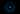Back to all Articles

# MSc. Research Project

#### by Vincent Ramdhanie 6 October 2019## Simulated Annealing

According to Wikipedia simulated annealing is

a generic probabilistic metaheuristic for the global optimization problem of locating a good approximation to the global optimum of a given function in a large search space.

Wait. What? Now you probably know less about simulated annealing than when you started. So let us see if this can be broken down a bit.

Suppose that you had a flat surface tray or dustbin cover and you gave it a few good whacks so that it became all uneven and puckered. Then suppose that you dropped a marble on the surface so that it rolled around a bit like a crazy roulette wheel. Eventually the marble will come to a stop in one of the indentations on the surface. However, it may not stop in the deepest indentation.

Suppose then that you wanted the marble to stop only in the deepest indentation and not any of the shallower ones. Initially the marble may have enough force to escape from some indentations. But as the force decreases the marble will have a harder and harder time escaping. Hopefully it escapes from the shallow ones but do not have enough force to escape from the deeper ones.

We can think of the action of the marble as searching for the deepest indentation. When it finds itself in an indentation, if it escapes then that was not the one it was looking for. If we can arrange that somehow the only one it becomes trapped in is the deepest one then we can say that it found the deepest indentation.

The only problem is how do we get it to find the deepest one?

Now say that everytime the marble comes to rest we can shake the tray a little so that the marble goes off in a random way. Then it settles again and we shake the tray again. But, each time we shake the tray we shake it a little gentler than the last. Eventually the shakes will be so gentle that the marble no longer escapes from the indentation that it is in.

The idea is that even the gentlest shake will get it out of the shallow indentation and hopefully into the one that we are looking for.

So my analogy may not be the best but it gets us thinking about local minimum (shallow indentations) and global minimum (the deepest indentation). We want to find the global. The algorithm is usually implementated in such a way that at each shake all the surrounding areas are considered. If they are higher than the current location of the marble then they are not considered unless the energy of the shake is enough to get it out. Of all the possible places the marble can end up after a shake the best one is choosen and the process repeats.

If we invert this idea we end up with the Hill Climbing Problem. In this case we are looking for the global maximum.

## CUDA

According to NVIDIA

CUDA® is a parallel computing platform and programming model invented by NVIDIA. It enables dramatic increases in computing performance by harnessing the power of the graphics processing unit (GPU).

The basic idea is to use many small processors working in parallel rather than one very powerful processor.

An excellent course on parallel programming using NVIDIA's CUDA can be found over at Udacity.

## Land Use Problem

There are K different land use types $\{k_1,k_2, ...,k_k\}$. For example Farm, School, Residential and so on. Each land use type must occupy a pre determined proportion of the total land area. Let $P_k$ be the proportion of land that must be allocated to land use $k$ where $k = 1, …, k$.

The total land area is divided into an $n \times m$ grid and each plot is associated with a set of land characteristics. Land characteristics may be water table, distance from city, forested, soil type, elevation, slope and so on. Each plot of land must have an associated value for each land characteristic. Suppose that there are r land characteristics. Let $L_r$ denote land characteristic $r$.

A plot of land is denoted by $G_{ij}$ where $i = 1, …, n$ and $j = 1, …, m$. Let $D_{ijk}$ denote a development cost for plot $G_{ij}$ for land use $K_k$. Each plot has a different development cost even for the same use as the characteristics affect the cost.

The intention is to maximize the value of the land at the lowest cost.

## One ring to bring them all

Now that we know that what can we do about it? I am attempting to implement the simulated annealing algorithm on the CUDA platform specifically to solve the land use problem.

## But First...Some Definitions

### Bibliography

Aerts, Jeroen C. J. H., and Gerard B. M. Heuvelink. "Using Simulated Annealing for Resource Allocation." International Journal of Geographical Information Science 16, no. 6 (12 2002): 571-87. doi:10.1080/13658810210138751.

Ferreiro, A. M., J. A. García, J. G. López-Salas, and C. Vázquez. "An Efficient Implementation of Parallel Simulated Annealing Algorithm in GPUs." Journal of Global Optimization 57, no. 3 (12 2013): 863-90. doi:10.1007/s10898-012-9979-z.

...